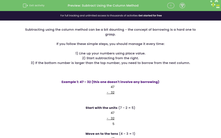# Subtract Using the Column Method

In this worksheet, students will practise using the column method in subtraction calculations using up to three digits and three numbers. This will include borrowing tens or hundreds.Key stage:  KS 4

Year:  GCSE

GCSE Subjects:   Maths

GCSE Boards:   Pearson Edexcel, OCR, Eduqas, AQA,

Curriculum topic:   Number, Number Operations and Integers

Curriculum subtopic:   Structure and Calculation Calculations with Integers

Popular topics:   Place Value worksheets

Difficulty level:#### Worksheet Overview

Subtracting using the column method can be a bit daunting - the concept of borrowing is a hard one to grasp.

If you follow these simple steps, you should manage it every time:

1) Line up your numbers using place value.

2) Start subtracting from the right.

3) If the bottom number is larger than the top number, you need to borrow from the next column.

Example 1: 47 - 32 (this one doesn't involve any borrowing)

 47 -   32

 47 -   32 5

Move on to the tens (4 - 3 = 1)

 47 -   32 15

Example 2: 843 - 269

 843 -   269

You can't do 3 - 9 without getting a negative so we need to borrow from the tens column.

If we take one ten away, we are left with 3 tens.

If we add this ten into the units column, we not have 13 to work with rather than 3.

We can write this process on the sum like this:

 8 313 -   2 6 9

We now have to calculate: 13 - 9 = 4

8 313
-   2 6 9
4

Move on to the tens

Once again we cannot do 3 - 6 so we must borrow, this time from the hundreds.

Here is how we show this on the sum:

 71313 -   2 6 9 4

We can now complete the sum: 13 - 6 = 7

 71313 -   2 6 9 7 4

Our final step is to subtract the hundreds

 71313 -   2 6 9 5 7 4

Before you start this activity, make sure you have a pen and paper handy so that you can write out your subtraction columns and sums.

### What is EdPlace?

We're your National Curriculum aligned online education content provider helping each child succeed in English, maths and science from year 1 to GCSE. With an EdPlace account you’ll be able to track and measure progress, helping each child achieve their best. We build confidence and attainment by personalising each child’s learning at a level that suits them.

Get started